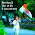## Saturday, 29 September 2018

### The Photoelectric Effect - Light as a Particle

In two of my previous posts, here and here, which talk about blackbody radiation and ultraviolet catastrophe, the radiation is described in terms of the physics of waves.  However, this is not the only way that light can be described. Consider the following...

In the above, light is being shone onto the metal plate (A) and a voltage (V) is applied between the the metal plate (A) and the cup (B). If the cup and the plate are made of the same material the the voltage measured by the volt meter is the same as the voltage between the plate and the cup. The light that is being shone onto the plate releases some electrons, and therefore the ammeter measures a current flowing. This effect is the photoelectric effect. The diagram of the apparatus may not be clear therefore is it worth pointing out that that there is no physical connection between the plate and the cup, hence the ammeter is measuring what is called a photoelectric current.

The above graph shows the typical results produced by such an aparatus, it shows the photoelectric current as a function of the applied voltage. The two lines on the graph represent the results obtained for two different intensities (brightness) of light shone upon the plate. In both set of results, if the voltage is made large enough the current reaches a maximum value and further increases in voltage produce no further increase in current. At this point the cup is collecting all of the electrons which are released by the plate.

If the voltage is reversed using the switch in the circuit some current will still flow, even through the voltage applied opposes the flow of the emitted electrons. This must mean that the electrons are released from the plate with an amount kinetic energy. However, it can be seen that if the reversed voltage is made large enough the current measured reduces to zero and it can also be seen that the voltage at which the current falls to zero is the same for both lines on the plot, where the two line represent results that would be obtained for two different light intensities. The voltage at which the current falls to zero, is called the stopping potential, and if this voltage is multiplied by the charge of an electron, the result gives the kinetic energy of the fastest electron emitted from the plate.

$$K_{max} = eV_0$$

This shows that the stopping potential is independent of the intensity of the light used. However, the stopping potential does depend upon the frequency of the light used. The representation below shows the typical behaviour found when the frequency of the light is varied and the stopping potential found

Therefore, for a given plate material, a linear relationship above a certain frequency is obtained, however, there is a cut off frequency below which no photoelectric effect occurs. This characteristic is produced no matter what the plate is made of.

There are three aspects of this effect that classical wave theory cannot explain:

1. Wave theory requires that as the intensity of the light increases so does its electrical fuel strength, E. If the lights electrical field strength increases then the force of the electron, eE. Thus, as intensity of the light increases, the force on the electron should get higher, an thus the kinetic energy which which the electron is emitted should get higher. However, this is at odds with the experimental results which suggests that the kinetic energy of the fasted electron emitted eV_nought is independent of the intensity of the light used.
2. According to wave theory, the photoelectric effect should occur no matter what frequency of light is used provided that the light is intense enough to eject the electron from the plate. However, the experimental results for any given surface there is  frequency below which the photoelectric effect does not occur, a cut-off frequency, no matter what intensity of light is used.
3. In classical theory the light energy is uniformly distributed across its wave front and the area over which this energy can be absorbed by the electron is a circle having an atomic diameter. Thus if the light of low intensity is used there should be a time delay between the moment that light is shined upon the plate and the production of current. That is, it should take the electron a measurable amount of time to absorb the light energy provided to it. However, no detectible time delay has ever been recorded while studying the photoelectric effect.
So it wave theory cannot explain these aspects of the photoelectric, what theory can?

Well, a very famous theoretical physicist came up with a theory (for which he surprisingly won the Nobel prize in physics although he is very much more well known for two of his other theories) that explains all of the aspects above, Albert Einstein, and it was his theory of the photon, a particle of light.  This other method that can be used to explain the behaviour of light will be the subject of my next post.

#### 1 comment:

1.if the impact of light on a non metal then what happen